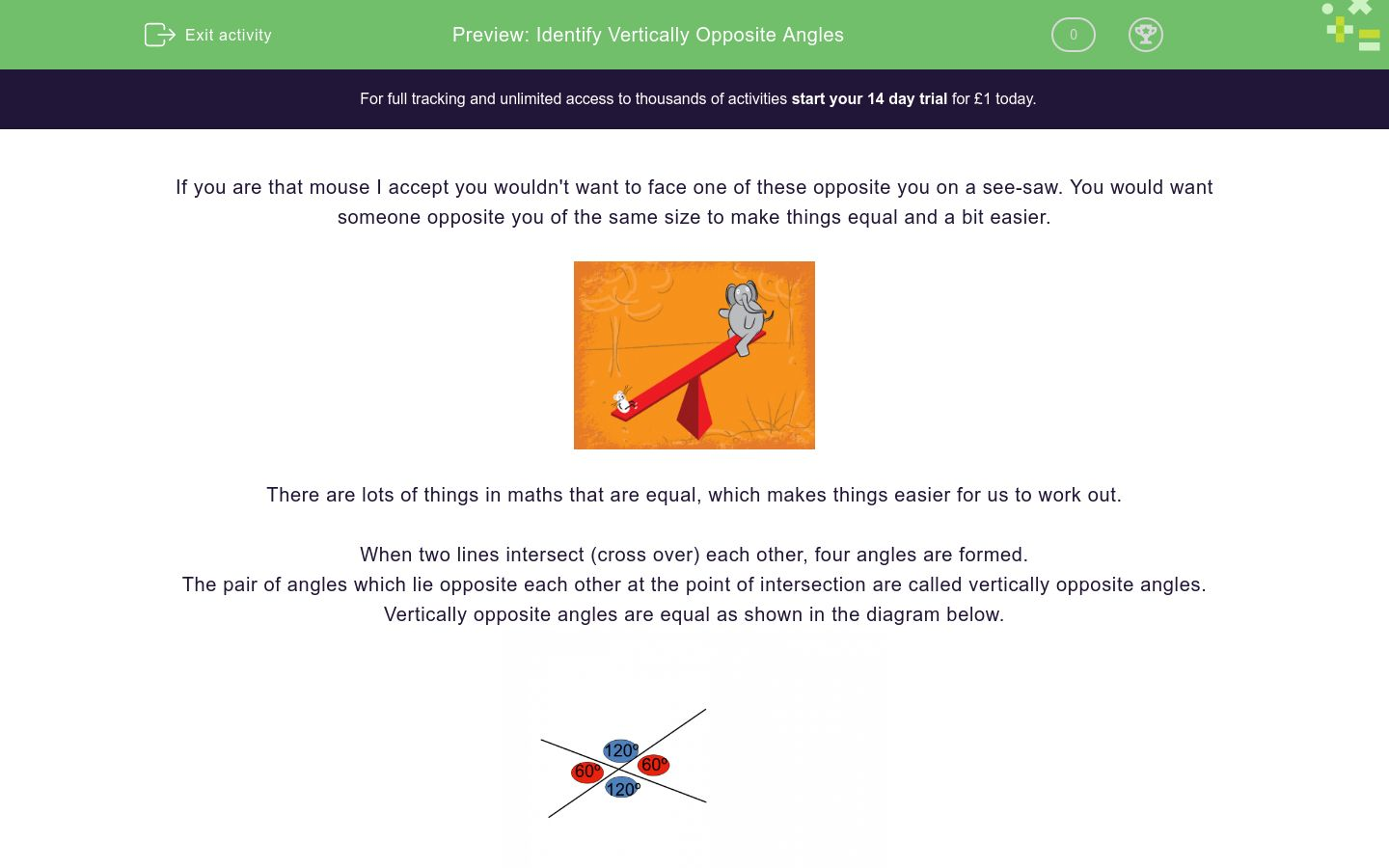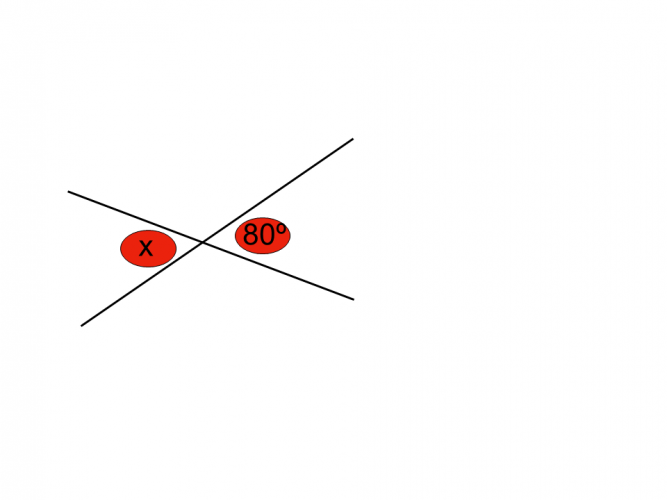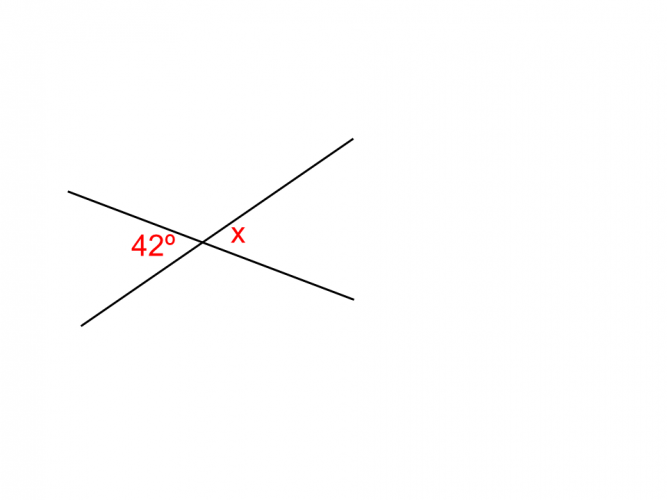# Identify Vertically Opposite Angles

In this worksheet, students will learn about vertically opposite angles and be able to apply the concept.Key stage:  KS 4

GCSE Subjects:   Maths

GCSE Boards:   AQA, Eduqas, Pearson Edexcel, OCR

Curriculum topic:   Geometry and Measures, Basic Geometry

Curriculum subtopic:   Properties and Constructions, Angles

Difficulty level:### QUESTION 1 of 10

If you are that mouse I except you wouldn't want to face one of these opposite you on a see saw. You would want someone opposite you of the same size to make things equal and a bit easier for us.There are lots of things in maths that are equal, which makes things easier for us.

When two lines intersect (cross over) each other, four angles are formed.

The pair of angles which lie opposite each other at the point of intersection are called vertically opposite angles.

Vertically opposite angles are equal as shown in the diagram below.As an added extra, can you see that the angles on the straight lines add up 180° another mathematical fact.

Let's see how you get onFrom the following list, choose the angles which are vertically opposite to each other.

b and f

a and c

h and f

d and hWhat is the value of x?ABC105° 98° 42° Graphic A x = Graphic B x = Graphic C x =What is the value of x?

125°

55°

35°

25°Calculate the value of x.

Write you answer in figures without the units.28° 98° 82° Value of x Value of y Value of Z28° 98° 82° Value of x Value of y Value of ZWhat is the value of x?

134°

92°

64°

46°From the following list, choose the angles which are vertically opposite to each other.

b and d

a and e

g and b

g and e

• Question 1From the following list, choose the angles which are vertically opposite to each other.

a and c
h and f
EDDIE SAYS
Find the point of intersection first, then they are easier to spot. Also you will notice that one angle is acute and the other obtuse. Just pick the ones that are either both acute or obtuse.
• Question 2What is the value of x?

80
EDDIE SAYS
80° is the correct answer as vertically opposite angles are equal. There is no working out to do which is always a bonus.
• Question 3EDDIE SAYS
Do you know the expression that opposites attract? They certainly do here. x is also 92°
• Question 4

ABC105° 98° 42° Graphic A x = Graphic B x = Graphic C x =
EDDIE SAYS
Are you singing the song a,b,c its as easy as 1,2,3...? Once you can spot the angles and know the rule you will be able to use it to solve other things.....
• Question 5What is the value of x?

25°
EDDIE SAYS
How did you find this one? You have all the knowledge you need to solve this. You know that 5 lots of x = 125° Just divide 125 by 5 to find 1 lot of x. 125 ÷ 5 = 25
• Question 6Calculate the value of x.

Write you answer in figures without the units.

12
EDDIE SAYS
Throw in algebra, not a problem for you. We know that 3 lots of x = 36, therefore 1 lot of x = 36 ÷ 3 = 12°
• Question 728° 98° 82° Value of x Value of y Value of Z
EDDIE SAYS
Here you are going to draw on other knowledge about angles. No problem for you though. You can see that y is vertically opposite to 28°, so that problem is solved. x, y(28°) and 54° add up to 180° for angles on a straight line. To find x 180 - 28- 54 = 98° x is vertically opposite to z making z 98°
• Question 8EDDIE SAYS
Quite often you have to identify angles that are not asked for in order to solve a problem. Here you need to put in the opposite angle in order to solve the rest. The opposite angle to 42° is also 42°. You know that angles on a straight line add up to 180° 180 -42 -87 = 51°
• Question 9What is the value of x?

46°
EDDIE SAYS
Did you spot this one? The opposite angle to 46° is 46° x is corresponding to the opposite 46° so is therefore also 46° because corresponding angles are also equal.
• Question 10From the following list, choose the angles which are vertically opposite to each other.

b and d
g and e
EDDIE SAYS
Sometimes you have loads of angles given all at one time and trying to remember which one is which can be tricky. Just find the point of intersection. See if the angle is acute or obtuse and look for the other acute or obtuse and you are good to go.
---- OR ----

Sign up for a £1 trial so you can track and measure your child's progress on this activity.

### What is EdPlace?

We're your National Curriculum aligned online education content provider helping each child succeed in English, maths and science from year 1 to GCSE. With an EdPlace account you’ll be able to track and measure progress, helping each child achieve their best. We build confidence and attainment by personalising each child’s learning at a level that suits them.

Get started